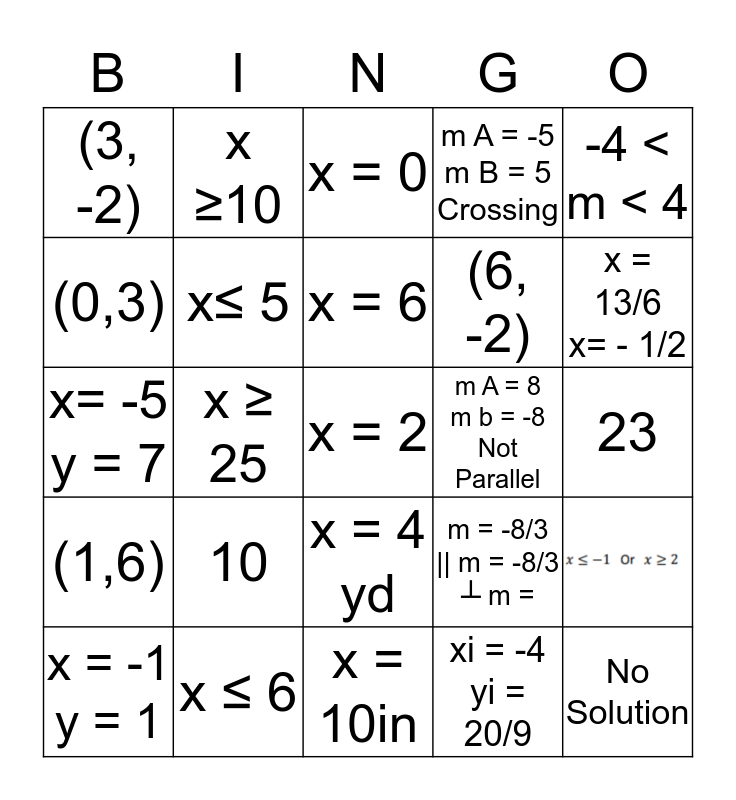# Q3 REVIEW: Linear, Inequalities, Systems of EqThis bingo card has 4 images and 46 words: (-7, -7), x ≤ 6, \$40 - x = Spent, (-4, 18), 23, (-1, 1), x ≥10, # min = 60h, (6, -2), -33, (3, -2), x≤ 5, x = 2, xi = -4 yi = 20/9, b> 20 or b < -4, x = 8 y = -4, x ≥ 25, x = 6, xi = 4 yi = -16, -4 < m < 4, x= -5 y = 7, Maximum of 7, x = 0, m A = 8 m b = -8 Not Parallel, (1,6), 10, x = 10, m A = -5 m B = 5 Crossing, x = 2 y = -7, 3G, n = - 1/2, m y = -2x + 23= -5, x = -1 y = 1, \$110.00, x = 10in, m = 3 yi = -6, x = 13/6 x= - 1/2, (7, -1), x≤ 30, x = 4 yd, ┴ m = 1/5, No Solution, (0,3), x ≥ 7, x = 6 and m = -8/3 || m = -8/3 ┴ m =.

⚠ This card has duplicate items: x = 6 (2)

## Play Online